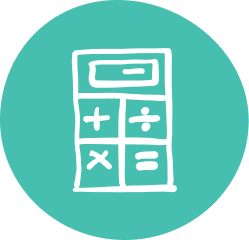### Quality Grade 11 Math Tutoring & Help

Oxford Learning®'s 11th Grade math tutoring program makes learning easy and fun, ensuring better math grades but, most importantly, ensuring a long term approach to learning.

By Grade 11, students should have a relatively clear idea of which path they want to take. Do they want to go to college or university, or head straight into the workplace? Whatever the path, Oxford Learning®’s Grade 11 math tutors help students get there.

Students receive quality instruction in Oxford Learning®’s Grade 11 math help program, helping them improve math comprehension. They also develop study skills, planning abilities, critical thinking skills, and confidence, as well as homework strategies to make math homework easier.

### Grade 11 Math Tutoring Subjects

##### Characteristics of Functions
• Understanding of functions
• Understanding functional representations
• Function inverses
##### Exponential Functions
• Evaluate powers with rational exponents
• Numeric, graphical, and algebraic representations of exponential functions
• Solve problems from real-world applications
##### Discrete Functions
• Recursive sequences
• Make connections between sequences, series, and financial applications
##### Trigonometric Functions
• Prove trigonometric identities
• Primary trigonometric ratios, the sine law, and the cosine law
• Expand and simplify quadratic expressions
• Solve problems involving quadratic functions
##### Exponential Functions
• Simplify and solve numerical expressions with exponents
• Compound interest and annuities
##### Trigonometric Functions
• Identify and represent sine functions
• Solve problems involving sine functions from real-world applications
##### Mathematical Models
• Learn to solve problems involving exponential relations from real-world applications
##### Personal Finance
• Compare different financial institutions and their services
• Relate compound interest to exponential growth
• Evaluate owning a vehicle
##### Geometry & Trigonometry
• Solve problems involving acute triangles using the sine law and cosine law with real-world applications
##### Data Management
• Collect data
• Organize data
• Analyze data